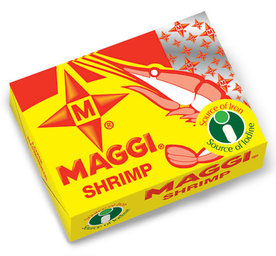# Third Term Examination Mathematics Primary 1 (Basic 1) – Exam Questions

MATHEMATICS

THIRD TERM EXAMINATION

PRIMARY 1 (BASIC 1)

SECTION A

Attempt all the questions.

Choose the correct answer from the options.

1. _____________ is a Nigeria currency.

[a] ₦5

[b] \$5

[c] €5

2. The smallest currency in Nigeria is _____________.

[a] ₦5

[b] 5k

[c] #5

3. 10k + 6k =

[a] 16k

[b] 15k

[c] 17k

4. Hand span can be used for measuring length of object.

[a] true

[b] false

[c] none of the above

5. l eat Lunch in _____________.

[a] morning

[b] afternoon

[c] night

6. l go to school in the _____________.

[a] morning

[b] afternoon

[c] night

7. ₦20 – ₦5 =

[a] ₦16

[b] ₦14

[c] ₦15

8. ₦35 + ₦5

[a] ₦45

[b] ₦46

[c] ₦40

9. Add these number together 14 + 12 + 13

[a] 40

[b] 38

[c] 39

10. _____________ is a natural unit use for measurement.

[a] Hand span

[b] Ruler

[c] Tape rule

What is the name of these shapes?11.

[a] Cube

[b] Cuboid

[c] Cylinder12.

[a] Sphere

[b] Cube

[c] Cylinder

13. Tick the shorter line.

[a] _________________

[b] __________________

[c] ________________

14. Tick the heavier object

[a] B

[b] b

[c] o

15. 12 divided by 2.

[a] 3

[b] 6

[c] 4

Get more Mathematics Exam Questions – Third Term Examination Mathematics Link

SECTION B

Attempt all questions in this section.

QUESTION 1

A. ₦4 + ₦10 + ₦1 =

B. 5k + 10k + 5k =

C. Add up 5k + 10 k =

D. ₦25 + ₦25 =

QUESTION 2

Calculate the change

Have               Spend             Change

A.       ₦50                 ₦25               ______

B.       10k                  1k                   ______

C.       ₦10                  ₦7                 ______

D.       ₦200                ₦150            ______

QUESTION 3

Estimate if these objects are heavy or light ; Tick for heavy, X for light.

Heavy or Light

A. House _____________

b. Flower _____________

c. Pencil _____________

d. Bell _____________

QUESTION 4

Draw the following shapes:

A. Circle

B. Triangle

C. Square

D. Rectangle

QUESTION 5

What says the time?

A                 B                C                DA. _____________

B. _____________

C. _____________

D. _____________